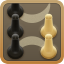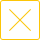# 24点数学游戏题目(包含数字2的数学游戏题目)

24点数学游戏题目，包含数字2的数学游戏题目，24点是一款比较适合孩子锻炼数学计算脑力以及团建活动的游戏，由于24点规则为利用四则运算凑成24点，所以需要玩家具备一定的数学运算能力，下面是24点数学游戏包含数字2的游戏题目及答案，希望可以帮到大家。2 2 2 3 :  (2+2)*2*3=24

2 2 2 4 :  (2+2+2)*4=24

2 2 2 5 :  (2*5+2)*2=24

2 2 2 7 :  (2*7-2)*2=24

2 2 2 8 :  (2+2)*(8-2)=24

2 2 2 9 :  (2+9)*2+2=24

2 2 2 10 :  2+2+2*10=24

2 2 3 3 :  (2+2)*(3+3)=24

2 2 3 4 :  (2+2+4)*3=24

2 2 3 5 :  (2*5-2)*3=24

2 2 3 6 :  (2/2+3)*6=24

2 2 3 7 :  (2/2+7)*3=24

2 2 3 8 :  (2-2+3)*8=24

2 2 3 9 :  (2+2)*(9-3)=24

2 2 3 10 :  (3+10)*2-2=24

2 2 4 4 :  (2*4-2)*4=24

2 2 4 5 :  2+2+4*5=24

2 2 4 6 :  (2-2+4)*6=24

2 2 4 7 :  4*7-(2+2)=24

2 2 4 8 :  (2+2)*4+8=24

2 2 4 9 :  2+4+2*9=24

2 2 4 10 :  (2+2)*(10-4)=24

2 2 5 5 :  5*5-2/2=24

2 2 5 6 :  (5-2/2)*6=24

2 2 5 7 :  2*5+2*7=24

2 2 5 8 :  (5+8)*2-2=24

2 2 5 9 :  (9-(2-5))*2=24

2 2 5 10 :  (2+5)*2+10=24

2 2 6 6 :  (2+6)/2*6=24

2 2 6 7 :  (2+7)*2+6=24

2 2 6 8 :  (8-(2+2))*6=24

2 2 6 9 :  (6/2+9)*2=24

2 2 6 10 :  2*10-(2-6)=24

2 2 7 7 :  (7-(2-7))*2=24

2 2 7 8 :  (7-(2+2))*8=24

2 2 7 10 :  (10/2+7)*2=24

2 2 8 8 :  (2+2)*8-8=24

2 2 8 9 :  2*9-(2-8)=24

2 2 8 10 :  2*8-2+10=24

2 2 9 10 :  10-(2-9)*2=24

2 2 10 10 :  2+2+10+10=24

2 3 3 3 :  (2+3+3)*3=24

2 3 3 5 :  (2+5)*3+3=24

2 3 3 6 :  (3-(2-3))*6=24

2 3 3 7 :  2*3*(7-3)=24

2 3 3 8 :  (2*3-3)*8=24

2 3 3 9 :  (2+3)*3+9=24

2 3 3 10 :  3*10-2*3=24

2 3 4 4 :  (2+3)*4+4=24

2 3 4 5 :  (5-(2-3))*4=24

2 3 4 6 :  (3-2)*4*6=24

2 3 4 7 :  (2-3+7)*4=24

2 3 4 8 :  (2-3+4)*8=24

2 3 4 9 :  2/3*4*9=24

2 3 4 10 :  3*10-(2+4)=24

2 3 5 5 :  2-3+5*5=24

2 3 5 6 :  (2-3+5)*6=24

2 3 5 7 :  3*7-(2-5)=24

2 3 5 8 :  2*8+3+5=24

2 3 5 9 :  2*3*(9-5)=24

2 3 5 10 :  (2+10)*(5-3)=24

2 3 6 6 :  (2+3)*6-6=24

2 3 6 7 :  6/2+3*7=24

2 3 6 8 :  (2+8)*3-6=24

2 3 6 9 :  (9-(2+3))*6=24

2 3 6 10 :  2*3*(10-6)=24

2 3 7 7 :  2*7+3+7=24

2 3 7 8 :  (2+7)/3*8=24

2 3 7 9 :  (7+9)/2/3=24

2 3 7 10 :  2*10-3+7=24

2 3 8 8 :  (8-(2+3))*8=24

2 3 8 9 :  (9-2*3)*8=24

2 3 8 10 :  2*3+8+10=24

2 3 9 9 :  2*3+9+9=24

2 3 9 10 :  2+3+9+10=24

2 3 10 10 :  10-(3-10)*2=24

2 4 4 4 :  (4-(2-4))*4=24

2 4 4 5 :  (2+5)*4-4=24

2 4 4 6 :  (2*4-4)*6=24

2 4 4 7 :  2*4*(7-4)=24

2 4 4 8 :  (2+4)*(8-4)=24

2 4 4 9 :  (9-2)*4-4=24

2 4 4 10 :  4-(2-4)*10=24

2 4 5 5 :  (5+5)*2+4=24

2 4 5 6 :  (2+4)*5-6=24

2 4 5 7 :  (5+7)/2/4=24

2 4 5 8 :  (2-4+5)*8=24

2 4 5 9 :  (2+4)*(9-5)=24

2 4 5 10 :  2*5+4+10=24

2 4 6 6 :  (2-4+6)*6=24

2 4 6 7 :  (2+6)*(7-4)=24

2 4 6 8 :  2/4*6*8=24

2 4 6 9 :  2*4*(9-6)=24

2 4 6 10 :  (2+4)*(10-6)=24

2 4 7 7：  (7+7)×2-4=24

2 4 7 8： 7*8/2-4=24

2 4 7 9： 2×4+7+9=24

2 4 8 8： 8+2*4+8=24

2 4 8 9： (9-(2+4))*8=24

2 4 9 9： 9+2+4+9=24

2 5 5 7： 5+5+2*7=24

2 5 5 8： (5/5+2)*8=24

2 5 5 9： 9+5+2*5=24

2 5 6 6： (2*5-6)*6=24

2 5 6 7： 7+5+2*6=24

2 5 6 8： 2+5*6-8=24

2 5 6 9： 9+5*6/2=24

2 5 7 7： 7+7+2*5=24

2 5 7 8： (2*5-7)8=24

2 5 7 9： 5*7-(2+9)=24

2 5 8 8： 5*8-2*8=24

2 5 8 9： 9+2+5+8=24

2 6 6 6： 6+6+2*6=24

2 6 6 7： (6+6*7)/2=24

2 6 6 8： (6/6+2)*8=24

2 6 6 9： (9-6)*(2+6)=24

2 6 7 8： (2+7-6)*8=24

2 6 7 9： 9+7+2+6=24

2 6 8 8： 8+2+6+8=24

2 6 8 9： (2*6-9)*8=24

2 6 9 9： 2*(9-6+9)=24

2 7 7 8： 8+7+2+7=24

2 7 8 8： (2+8-7)*8=24

2 7 8 9： 2*(7+9)-8=24

2 8 8 8： (8/8+2)*8=24

2 8 8 9： (2+9-8)*8=24

2 8 9 9： (9/9+2)*8=24

## 精品排行

1189759人在玩 2
###### 常州四副牌​（四副头）
23201人在玩 3
###### 双陆棋
3## 24点热门攻略更多